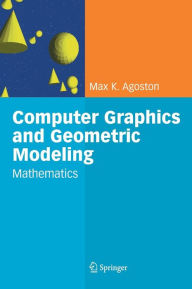# Computer Graphics and Geometric Modelling: Mathematics Max K. Agoston AuthorBrand: Springer London
109.99 USD

Possibly the most comprehensive overview of computer graphics as seen in the context of geometric modelling, this two volume work covers implementation and theory in a thorough and systematic fashion. Computer Graphics and Geometric Modelling: Mathematics, contains the mathematical background needed for the geometric modeling topics in computer graphics covered in the first volume. This volume begins with material from linear algebra and a discussion of the transformations in affine & projective geometry, followed by topics from advanced calculus & chapters on general topology, combinatorial topology, algebraic topology, differential topology, differential geometry, and finally algebraic geometry. Two important goals throughout were to explain the material thoroughly, and to make it self-contained. This volume by itself would make a good mathematics reference book, in particular for practitioners in the field of geometric modelling. Due to its broad coverage and emphasis on explanation it could be used as a text for introductory mathematics courses on some of the covered topics, such as topology (general, combinatorial, algebraic, and differential) and geometry (differential & algebraic).